Towards a Better Understanding of Pi
Using the Idea of Limits.

Ed Mickleburgh
Student in the GDipEd Program
University of Canberra, 1997
edmickle@ozemail.com.au

This article shows a way to increase students' appreciation of what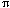represents and offers some activities that will enable them to arrive at their own approximations ofwithin bounded limits. To use the methods that follow, your students will need to know the basic trigonometric ratios. They will also need to use the trigonometric function keys on a calculator. By building on these skills, the activities might provide a good preparation for the the idea of limits in a pre-calculus context. An optional activity requires a computer with a fairly basic spreadsheet application.
The motivation for this article comes out of some responses to the question 'what is?'. Some students seem to think it is merely a number which is used to calculate the area of a circle or its circumference. One mature-age student had been told in the pre-calculator days thatwas 22/7, and that was that! Often,seems to be 'defined' as a calculator key. Not often enough, perhaps, isseen for what it is: the exact ratio of the circumference (C) of a circle to its diameter (d).
At all events, it doesn't seem to be widely recognised thatis an extremely important number which has many interesting properties and is deserving of study in its own right.

Adapting Archimedes

I believe a good way to get an 'intuitive feel' for the fact that= C/d is by following the reasoning used by Archimedes who developed the first scientific method for calculating in about 240BC. [You may wish students to construct some or all of the following diagrams with a compass and protractor or you may prefer to use prepared overhead transparencies.]
Archimedes' reasoning went roughly like this:
 if you take a circle and circumscribe it with hexagon, then the perimeter of the hexagon will be longer than the circumference of the interior circle - figure 1(a) if you were to take the same circle and inscribe a hexagon within it, then the perimeter will be less than the circumference in length - figure 1(b)
From the figures, students should realise that the length of the circumference of the circle will be somewhere between the lengths of the two perimeters.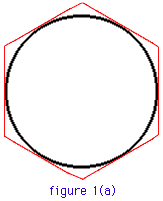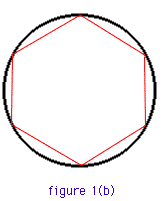Archimedes went on to consider what would happen if the number of sides of the polygon increased from six to twelve - figures 2(a) and 2(b).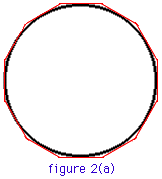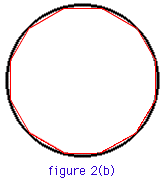Students should have no problems in seeing that: the perimeter lengths of the circumscribed and inscribed dodecagons are shorter and longer respectively than those of the preceeding hexagons both perimeters are getting closer to the circumference and that the length of the circumference is of course somewhere between the lengths of the two perimeters - as with the hexagons. The following diagrams summarise the idea and may add clarification: figure 3(a) shows hexagons circumscribed about and inscribed within a circle and figure 3(b) shows the same in respect of the dodecagons.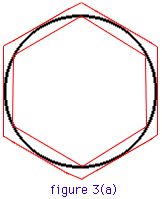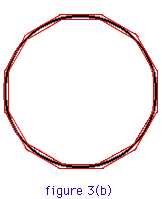This is where we modify the approach taken by Archimedes, because his method of progressively doubling the number of sides of the polygons to 96, is rather too complex for classroom use. Of course, he didn't have the use of the trigonometric functions that are about to be used here and which speed-up the process considerably. Those wishing to see how Archimedes' estimated are referred to Heath (1963).
The bottom line is that by expressing the perimeters of the 96-sided polygons to the diameter of the circle, he knew that:

223/71 << 22/7.

We start by calculating the lengths of one side of a polygon. Students should begin by constructing two circles of the same arbitrary radius which can be set to r = 1. By using a protractor, hexagons can be readily constructed in the following fashion - figures 4(a) and (b).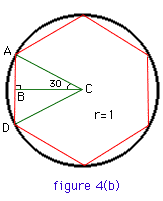Students might first consider the circumsrcibed hexagon in figure 4(a). By drawing a line from the centre C to the vertex A and another from C to the mid-point of AD which is labelled B, it should be clear that triangle ABC is right-angled. It should also be clear that angle ACB is 30 degrees. Can students now see that if we can find AB, we have found the length of half the side of a hexagon? They should be able to work out that:
 tan(60/2) = tan(30) = AB / 1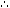AB = tan(60/2)perimeter of circumscribed polygon = 2 x 6 x tan(60/2)= C/d < 12 tan(60/2)/2< 3(2) tan(60/2).
The class should then draw the diagram shown in figure 4(b) above to consider the case of the inscribed polygon. In a way similar to that just shown, students should see that the half-length of a side to the hexagon is AB, and so:
 sin(60/2) = sin(30) = AB / 1AB = sin(60/2)perimeter of inscribed polygon = 2 x 6 x sin(60/2)= C/d > 12 sin(60/2)/2> 3(2) sin(60/2).
So now we have a bounded limit for3(2) sin(60/2) << 3(2) tan(60/2).

Now we want to double the number of sides to the polygons so that we get a better approximation of the circumference. Students should quickly grasp that this is done by bisecting the angle ACB in each case - figures 5(a) and (b).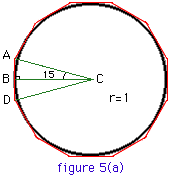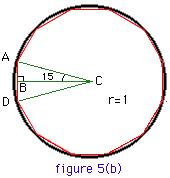It is easy to show that by following the steps for the case of the hexagon, we can get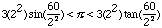For example for the circumscribed polygon - figure 5(a)

 tan(60/4) = tan(15) = AB / 1AB = tan(60/4)perimeter of circumscribed polygon = 2 x 12 x tan(60/4)= C/d < 12 tan(60/4)/2< 3(2x2) tan(60/(2x2)).
Incidentally, does this process suggest that a circle is a polygon with an infinite number of sides?

Sequences

By now it may have occurred to some students, at least those who have some knowledge of sequences and series, that two sequences emerge from the continuous doubling of the number of the sides and the bisection of the angles. The number of sides of the polygons are

6 = 3(2), 12 = 3(2x2), 24 = 3(2x2x2), 48 = 3(2x2x2x2),...

and the angles are

30 = 60/2, 15 = 60/(2x2), 7.5 = 60/(2x2x2), 3.75 = 60/(2x2x2x2),...

Thus, we can state that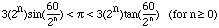--- (*)

With this limit-bounded formula, students could of course play around with their calculators to get various approximations of . If your class has access to a standard spreadsheet, you may wish to try the following activity.

Using Computers

It is simple enough to use a standard spreadsheet to get a table of successive approximations of.

No. Sidesn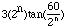Rel. ErrorRel. Error
613.46410210.2657793.000000-4.507034
1223.2153902.3490523.105829-1.138407
24 3 3.159660 0.575100 3.132629 -0.285334
48 4 3.146086 0.143035 3.139350 -0.071379
96 5 3.142715 0.035713 3.141032 -0.017848
192 6 3.141873 0.008925 3.141452 -0.004462
384 7 3.141663 0.002231 3.141558 -0.001116
768 8 3.141610 0.000558 3.141584 -0.000279
1536 9 3.141597 0.000139 3.141590 -0.000070

At this point, students could reflect on:

which side of the limit, 'tan' or 'sin' boundaries, starts-off as a better approximation tothe rates at which they appear to converge towardsArchimede's limit for the 96-sided polygon given above. Plotting the data might assist: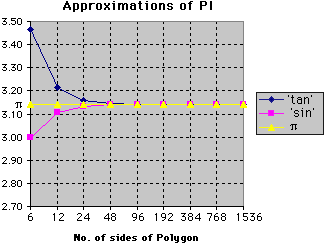Conclusion

The purpose of this article was to heighten students' appreciation ofas an irrational number by first demonstrating that the circumference of a circle can be approximated by considering the inscription and circumscription of a series of polygons. Students will then see that by expressing these approximations as a ratio to a diameter,exists within bounded limits and hence can be approximated as accuracately as we please (as Archimedes himself might have said).
The history ofis quite fascinating and covers a wide range of mathematical concepts. There is a lighter side to its history as well which can be used to good effect in the classroom.

Note:

Students learning calculus might find the inequatities (*) interesting in another situation. Dividing through the inequalities (*) bygives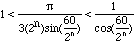If the angle is expressed in radians rather than degrees these inequalities can be written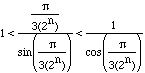Hence, as n increases,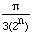approaches 0 and hence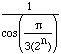approaches 1.

Thus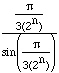is sandwiched between 1 and a sequence that converges to 1, giving some justification to the fact that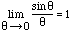References

 Eves H (1990) An Introduction to the History of Mathematics Orlando, FL: Sanders College Heath T (1963) Greek Mathematics New York: Dover Katz VJ (1993) A History of Mathematics: An Introduction New York: Harper CollinsReturn to Math Central

To return to the previous page use your browser's back button.